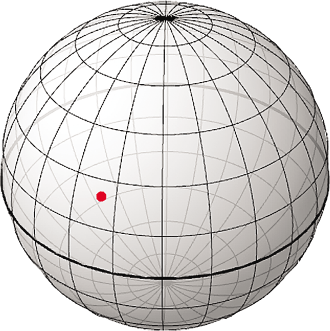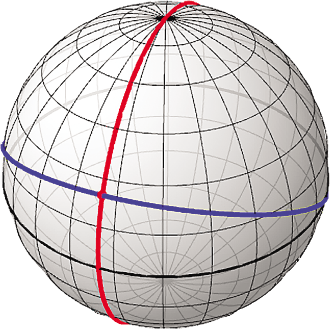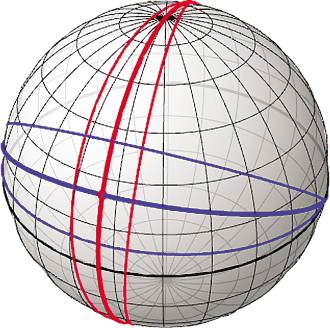September 2012

Volume 27 Number 09

# Touch and Go - Exploring Spherical Coordinates on Windows Phone

By Charles Petzold | September 2012Ever since I became acquainted with the Motion sensor in Windows Phone 7.1, I’ve known exactly what I wanted to do with it: resurrect some C# positional astronomy code I wrote about five years ago and wire it up with Motion sensor logic into a Windows Phone program. Such a program would allow me to point the phone at a spot in the night sky to show the planets and constellations located there.

That program—with the ridiculous name AstroPhone—is coming in the next installment of this column. Meanwhile, some conceptual, mathematical and programmatic groundwork is necessary.

As I’ve been discussing in previous columns, the Motion sensor consolidates input from the compass, accelerometer, gyroscope and GPS, and computes (among other things) a 3D rotation matrix that describes the phone’s orientation in space. It’s convenient to apply this matrix to a 3D vector relative to the phone’s coordinate system. This transformed vector points to a location on the conceptual celestial sphere. A location on this sphere is a horizontal coordinate, which consists of an altitude—an angle above or below the viewer’s horizon—and an azimuth, which is basically a compass direction.

Horizontal coordinates are analogous to the familiar geographic coordinates that identify a position on the surface of the Earth. The altitude above or below the viewer’s horizon is analogous to a latitude above or below the Earth’s equator. The azimuth indicating a compass direction around the viewer’s horizon is analogous to the longitude around the Earth’s equator. As you’ll see, all types of spherical coordinates are fundamentally the same, differing most crucially by the plane that separates the upper hemisphere from the lower.

## Flattening the Sphere

In last month’s column (msdn.microsoft.com/magazine/jj553520), I demonstrated how to use horizontal coordinates to scan large photographs and other images by changing the phone’s orientation in space. However, that program “cheated” a bit by simply ignoring the true nature of spherical coordinates. It refused to acknowledge the fact that circles of latitude become increasingly smaller toward the top and bottom of the celestial sphere, and consequently, degrees of azimuth become increasingly compressed.

A program that provides a simulated viewing of the night sky simply can’t take those shortcuts. It must find an algorithmic way to map an area of the celestial sphere to the flat screen of the phone. Of course, flattening the sphere has been a problem encountered by mapmakers for centuries. It can’t be done without distortion, but the objective is to minimize those distortions.

Figure 1 shows a sphere decorated with lines of altitude and azimuth every 15°. From a vantage point at the center of the inside of the sphere, the red dot represents a point that you’re viewing or pointing at with your phone. The altitude of this particular point is 25°. The azimuth depends on where you start counting and in what direction.Figure 1 A Point (in Red) on a Celestial Sphere

Let’s call this point the “view center” because it’s the point that will be mapped to the center of the phone’s screen. What lines on the sphere correspond to the edges of the screen?

It’s tempting to assume that the edges of the phone’s screen will correspond to lines of altitude and azimuth. But that only seems reasonable at locations near the horizon. The concept starts to break down as the view center gets further from the horizon, and collapses altogether when you’re viewing straight up or down at the poles.

Instead, let’s construct orthogonal “great circles” at the view center, as shown in Figure 2.Figure 2 Orthogonal Great Circles Crossing the View Center

Great circles are an important concept in spherical geometry. A great circle is a two-dimensional circle in 3D space sharing the same radius and center as the sphere itself. Great circles on the sphere are analogous to straight lines on a plane because they represent the shortest distance between two points. Lines of azimuth (and longitude) are great circles. Lines of altitude (and latitude) are not; they are instead “small circles,” except for the circle that divides the sphere in two—the horizon (or equator).

The red great circle in Figure 2 passes through the two poles and is simply an azimuth line. The blue great circle crosses the horizon at azimuths 90° from the azimuth of the view center.

A rectangle surrounding that view center will be mapped to the phone’s screen. Conceptually, this rectangle can be a grid of great circles. Let’s construct two more great circles in blue that cross the horizon at the same point as the first one. And let’s construct two more red great circles that also share two points with the first one. The result is shown in Figure 3. The shared points of the red great circles have the same azimuth as the view center (or the azimuth plus 180°) but the altitude is offset by 90°.Figure 3 Additional Great Circles to Create a Rectangular Area

Those additional great circles define the edges of a rectangle that corresponds to the portrait-mode screen of the phone, with a width of about 19° of arc and a height of 32° of arc. If you assume that the phone’s screen is about 2 inches wide and 3.33 inches high, these arc degrees are appropriate when the phone’s screen is held about 6 inches from your face. The phone’s screen is 480 pixels wide and 800 pixels high, so these numbers also imply 25 pixels per arc degree.

When implementing this projection scheme in an algorithm, you need to work backward: Think of the two points shared by the three blue great circles as an axis, and the two points shared by the three red great circles as another axis. Together with the vector to the view center, these form an orthonormal coordinate system.

Vector algebra allows deriving angle offsets of any other coordinate from the view center, as shown in the HorizontalCoordinateProjection class in Figure 4. The class has one method to set the view center, and another method to obtain angle offsets of the horizontal coordinate of any other object relative to that view center.

Figure 4 The HorizontalCoordinateProjection Class

``````public class HorizontalCoordinateProjection
{
Vector3 viewCenterVector, horzAxis, vertAxis;
public void SetViewCenter(HorizontalCoordinate viewCenterCoord)
{
viewCenterVector = viewCenterCoord.ToVector();
HorizontalCoordinate vertAxisCoord =
new HorizontalCoordinate(viewCenterCoord.Azimuth + 90, 0);
vertAxis = vertAxisCoord.ToVector();
horzAxis = Vector3.Cross(viewCenterVector, vertAxis);
}
public void GetAngleOffsets(HorizontalCoordinate objectCoord,
out double horzAngle, out double vertAngle)
{
Vector3 objectVector = objectCoord.ToVector();
Vector3 horzObjectCross = Vector3.Cross(objectVector, -horzAxis);
Vector3 vertObjectCross = Vector3.Cross(objectVector, vertAxis);
horzObjectCross.Normalize();
vertObjectCross.Normalize();
double x = Vector3.Dot(horzObjectCross, vertAxis);
double y = Vector3.Dot(horzObjectCross, viewCenterVector);
horzAngle = -180 * Math.Atan2(y, x) / Math.PI;
x = Vector3.Dot(vertObjectCross, horzAxis);
y = Vector3.Dot(vertObjectCross, viewCenterVector);
vertAngle = -180 * Math.Atan2(y, x) / Math.PI;
}
}
``````

To determine where that object should be positioned on the phone’s screen, the angles obtained from the GetAngleOffsets method need to be multiplied by a constant you select for the number of pixels per arc degree. I suggested earlier that this constant should equal 25 when the phone is held 6 inches from your face, but you might want to go with something lower to provide a broader view.

## Viewing the Sphere from the Inside

The ViewHorizontalCoordinates program sets its PIXELS_PER_DEGREE constant to 15 to display horizontal coordinates from the inside looking out, as shown in Figure 5. That particular view occurs when the phone points a little south of east, a bit above the horizon.Figure 5 The ViewHorizontalCoordinates Program

For each CurrentValueChanged event fired by the Motion sensor, the event handler begins by obtaining the rotation matrix that indicates how the Earth is oriented relative to the phone. It converts that to a HorizontalCoordinate value, and sets the view center in a previously created HorizontalCoordinateProjection object:

``````Microsoft.Xna.Framework.Matrix matrix =
HorizontalCoordinate viewCenter =
HorizontalCoordinate.FromMotionMatrix(matrix);
coordinateProjection.SetViewCenter(viewCenter);
rotate.Angle = -viewCenter.Tilt;
``````

The rotate object is a RotateTransform applied to the entire Canvas. The handler then implements several loops involving altitude and azimuth values in 15° increments. The first time the CurrentValueChanged event fires, the event handler creates all the necessary Line and TextBlock elements and adds them to the Canvas. The second and subsequent times through, the handler simply accesses the existing Line and TextBlock elements already in the Canvas and sets new points.

Each HorizontalCoordinate value needs to be converted to a screen coordinate. That’s the job of the CalculateScreenPoint method in Figure 6, which calls the GetAngleOffsets method in HorizontalCoordinateProjection and multiplies the angles by the PIXELS_PER_DEGREE constant.

Figure 6 Calculating a Screen Point from a Horizontal Coordinate

``````Point CalculateScreenPoint(HorizontalCoordinate horizontalCoordinate)
{
double horzAngle, vertAngle;
coordinateProjection.GetAngleOffsets(horizontalCoordinate,
out horzAngle, out vertAngle);
// Use NaN to indicate points clearly out of range of the screen
float x = float.NaN;
float y = float.NaN;
if (horzAngle > -90 && horzAngle < 90 &&
vertAngle > -90 && vertAngle < 90)
{
x = (float)(width / 2 + PIXELS_PER_DEGREE * horzAngle);
y = (float)(height / 2 + PIXELS_PER_DEGREE * vertAngle);
}
return new Point(x, y);
}
``````

The GetAngleOffsets method always returns angles in the range -180° to 180°. Sometimes lines straddle these limits and this creates a wraparound problem. For example, a line might (in theory) extend from -175° to 175°. That line should be only 10° in length, but the calculated length would be 380°! The logic in CalculateScreenPoint involving NaN (“not a number”) corrects this problem by flagging all points with angle offsets less than -90° or greater than 90°, which is out of the range of the screen.

I wanted the text labels showing the altitude angles to be visible regardless of the azimuth, and those showing the compass points to be visible no matter how high or low you point the phone. The altitude labels are displayed with a screen point calculated from the view center azimuth, and the compass labels are displayed with a screen point calculated from the view center altitude, with a little adjustment so they don’t all cluster together when you point the phone straight up or down. This little trick helps keep the labels inside the screen, perhaps rotated a bit away from the center.

## A Switch to XNA

As I was working on the programs for this column in conjunction with AstroPhone for next month, I started to notice some performance issues. The ViewHorizontalCoordinates program can only manage about 10 frames per second on my development phone, and the problem seems to be more in the Silverlight layout system rather than in my code.

With some reluctance I decided that the remaining programs would target the Windows Phone XNA framework rather than Silverlight. This is the case for the ViewThreeCoordinates project, which is similar to ViewHorizontalCoordinates but written for XNA rather than Silverlight.

## Library Preview

The ViewThreeCoordinates project also reveals some of the strategies I’ll be using for the full-blown astronomy program. It makes use of part of a larger library called Petzold.Astronomy. In constructing this library, my primary reference has been the second edition of the classic book “Astronomical Algorithms” by Jean Meeus (Willmann-Bell, 1998).

Positional astronomy requires a lot of trigonometry. In the Petzold.Astronomy library, I try to avoid confusion and conversions between degrees and radians by implementing a struct named Angle. You can create an Angle value from either a degree or a radian using the static FromDegree or FromRadian methods, and get the degrees or radians out, but it’s not often necessary because Angle also implements all the necessary trigonometric functions as properties.

For example, if you have an Angle value named myAngle, you can obtain the cosine of that angle like so:

``````double x = myAngle.Cosine;
``````

If you think of sines, cosines and tangents as “properties” of angles, this makes perfect sense. But notice how the Cosine property is implemented:

``````public double Cosine
{
set { radians = Math.Acos(value); }
}
``````

The set accessor is the inverse cosine function, which means you can set an existing Angle value to the inverse cosine of a number using a statement like this:

``````myAngle.Cosine = x;
``````

The only place where this process didn’t quite work was in implementing the essential Atan2 method. I did it with a static method that creates an Angle value:

``````public static Angle ArcTangent(double y, double x)
{
}
``````

## Equatorial Coordinates

The ViewThreeCoordinates program displays horizontal coordinates in green, plus two other coordinate systems very useful in positional astronomy: equatorial coordinates in blue and ecliptic coordinates in red. Figure 7shows a typical display with the phone pointing north and an altitude of 25°. (Yes, I know the screen is cluttered, but you can tap the screen to display only one or two sets of coordinates.)Figure 7 The ViewThreeCoordinates Display

As the Earth rotates on its axis and revolves around the sun, the Earth’s equator stays pretty much in the same plane. The plane of the Earth’s equator is the fundamental plane associated with equatorial coordinates. Equatorial coordinates consist of a declination—positive above the equator and negative below—and a right ascension going around the equator. The right ascension is usually specified in hours rather than degrees, where each hour is equivalent to 15°.

Of course, geographic coordinates (longitude and latitude) are also based on the Earth’s equator, but geographic coordinates rotate with the Earth while equatorial coordinates are fixed relative to the rest of the universe, and hence seem to turn as the Earth rotates on its axis. The positions of stars are specified in equatorial coordinates, and they don’t change much with the passage of time.

ViewThreeCoordinates displays a grid of equatorial coordinates by converting them to horizontal coordinates. This conversion depends on the observer’s geographic location (longitude and latitude) and the current time, and is implemented in the HorizontalCoordinate structure.

However, a very rough conversion of right ascension to azimuth is possible: At a local time of midnight on the vernal equinox (March 20 or thereabouts), 0 hours right ascension is north (0° of azimuth). The bright star Arcturus has a right ascension of about 14 hours, so on that date at that time, you’ll need to swing westward by 210° (or eastward by 150°) to see it. Or, you can just wait two hours until 2 a.m. and Arcturus will be due south.

You can calculate the local hour angle of a star by subtracting its right ascension from the number of hours since midnight local time. Convert to degrees by multiplying by 15, and add the number of days since March 21, and that’s the azimuth of the star measured eastward from north.

If you run ViewThreeCoordinates and hold the phone up to the night sky, the northern equatorial pole (visible at the very top of Figure 7) will correspond with the location of Polaris, the North Star, which has a declination of very nearly 90° and hence coincides with the vector of the Earth’s axis. Notice how the azimuth line of North intersects that pole.

## Ecliptic Coordinates

The orbits of all the planets of the solar system are roughly in the same plane. This plane is called the ecliptic, and it’s the basis of ecliptic coordinates (also known as celestial coordinates), which are displayed by ViewThreeCoordinates in red. An ecliptic coordinate consists of an ecliptic longitude and ecliptic latitude.

Ecliptic coordinates are mostly used when computing the positions of the sun, planets and moon. Because the sun and planets lie in roughly the same plane, the ecliptic latitude of these objects is usually close to zero. The ecliptic itself is displayed with a thick red line in ViewThreeCoordinates. If you hold up the phone to the day or night sky, the sun and planets should coincide with that line.

Equatorial coordinates and ecliptic coordinates differ solely as a result of the Earth’s tilt of about 23° relative to the ecliptic. An equatorial coordinate of 0 hours right ascension and 0° declination is the same as an equatorial coordinate of 0° longitude and 0° latitude, and the two systems also coincide at 180°.

On the vernal equinox, the sun has an ecliptic longitude of 0°. That longitude increases by approximately 1° per day over the course of the year. Hence, the ecliptic longitude of the sun in degrees is approximately equal to the number of days since March 21.

Ecliptic longitudes are often labeled with the signs of the zodiac, starting with Aries for 0°-30°, Taurus for 30°-60°, Gemini for 60°-90° and so forth through Pisces for 330°-360°. I have also done this in ViewThreeCoordinates. These signs of the zodiac are constellations that are approximately located at these ecliptic longitudes with ecliptic latitudes in the neighborhood of zero. Thus, the sun is “in Aries” (meaning that Aries is beyond the sun) during the month beginning March 21.

Enough theory. In the next appearance of this column, the grid lines of the spherical coordinate systems will be replaced with the sun, moon, planets, stars and constellations.

Charles Petzold is a longtime contributor to MSDN Magazine, and is currently updating his classic book “Programming Windows” (Microsoft Press, 1998) for Windows 8. His Web site is charlespetzold.com.

Thanks to the following technical expert for reviewing this article: Donn Morse